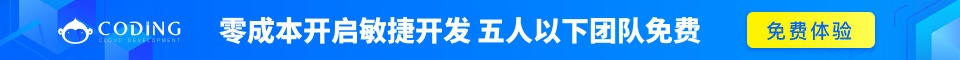# jQuery UI 如何使用部件库

```\$.widget( "custom.progressbar", {
_create: function() {
var progress = this.options.value + "%";
this.element
.text( progress );
}
});
```

```\$( "<div></div>" )
.appendTo( "body" )
.progressbar({ value: 20 });
```

```\$.widget( "custom.progressbar", {

// Default options.
options: {
value: 0
},
_create: function() {
var progress = this.options.value + "%";
this.element
.text( progress );
}
});
```

### 调用插件方法

```\$.widget( "custom.progressbar", {

options: {
value: 0
},

_create: function() {
var progress = this.options.value + "%";
this.element
.text( progress );
},

// Create a public method.
value: function( value ) {

// No value passed, act as a getter.
if ( value === undefined ) {
return this.options.value;
}

// Value passed, act as a setter.
this.options.value = this._constrain( value );
var progress = this.options.value + "%";
this.element.text( progress );
},

// Create a private method.
_constrain: function( value ) {
if ( value > 100 ) {
value = 100;
}
if ( value < 0 ) {
value = 0;
}
return value;
}
});
```

```var bar = \$( "<div></div>" )
.appendTo( "body" )
.progressbar({ value: 20 });

// Get the current value.

// Update the value.
bar.progressbar( "value", 50 );

// Get the current value again.
```

### 使用选项

`option()` 方法是自动提供给插件的。`option()` 方法允许您在初始化后获取并设置选项。该方法像 jQuery 的 `.css()``.attr()` 方法：您可以只传递一个名称作为取值器来使用，也可以传递一个名称和值作为设置器使用，或者传递一个键名/键值对的哈希来设置多个值。当作为取值器使用时，插件将返回与传入名称相对应的选项的当前值。当作为设置器使用时，插件的 `_setOption` 方法将被每个被设置的选项调用。我们可以在我们的插件中指定一个 `_setOption` 方法来反应选项更改。对于更改选项要独立执行的动作，我们可以重载 `_setOptions`

```\$.widget( "custom.progressbar", {
options: {
value: 0
},
_create: function() {
this.options.value = this._constrain(this.options.value);
this.refresh();
},
_setOption: function( key, value ) {
if ( key === "value" ) {
value = this._constrain( value );
}
this._super( key, value );
},
_setOptions: function( options ) {
this._super( options );
this.refresh();
},
refresh: function() {
var progress = this.options.value + "%";
this.element.text( progress );
},
_constrain: function( value ) {
if ( value > 100 ) {
value = 100;
}
if ( value < 0 ) {
value = 0;
}
return value;
}
});
```

### 添加回调

```\$.widget( "custom.progressbar", {
options: {
value: 0
},
_create: function() {
this.options.value = this._constrain(this.options.value);
this.refresh();
},
_setOption: function( key, value ) {
if ( key === "value" ) {
value = this._constrain( value );
}
this._super( key, value );
},
_setOptions: function( options ) {
this._super( options );
this.refresh();
},
refresh: function() {
var progress = this.options.value + "%";
this.element.text( progress );
if ( this.options.value == 100 ) {
this._trigger( "complete", null, { value: 100 } );
}
},
_constrain: function( value ) {
if ( value > 100 ) {
value = 100;
}
if ( value < 0 ) {
value = 0;
}
return value;
}
});
```

```var bar = \$( "<div></div>" )
.appendTo( "body" )
.progressbar({
complete: function( event, data ) {
}
})
.bind( "progressbarcomplete", function( event, data ) {
alert( "Events bubble and support many handlers for extreme flexibility." );
alert( "The progress bar value is " + data.value );
});

bar.progressbar( "option", "value", 100 );
```

## 本质

```var bar = \$( "<div></div>" )
.appendTo( "body" )
.progressbar()
.data( "progressbar" );

// Call a method directly on the plugin instance.
bar.option( "value", 50 );

// Access properties on the plugin instance.
```

```var bar = \$.custom.progressbar( {}, \$( "<div></div>" ).appendTo( "body") );

// Same result as before.
```

### 扩展插件的原型

```\$.custom.progressbar.prototype.reset = function() {
this._setOption( "value", 0 );
};
```

### 清理

```\$.widget( "custom.progressbar", {
options: {
value: 0
},
_create: function() {
this.options.value = this._constrain(this.options.value);
this.refresh();
},
_setOption: function( key, value ) {
if ( key === "value" ) {
value = this._constrain( value );
}
this._super( key, value );
},
_setOptions: function( options ) {
this._super( options );
this.refresh();
},
refresh: function() {
var progress = this.options.value + "%";
this.element.text( progress );
if ( this.options.value == 100 ) {
this._trigger( "complete", null, { value: 100 } );
}
},
_constrain: function( value ) {
if ( value > 100 ) {
value = 100;
}
if ( value < 0 ) {
value = 0;
}
return value;
},
_destroy: function() {
this.element
.removeClass( "progressbar" )
.text( "" );
}
});
```